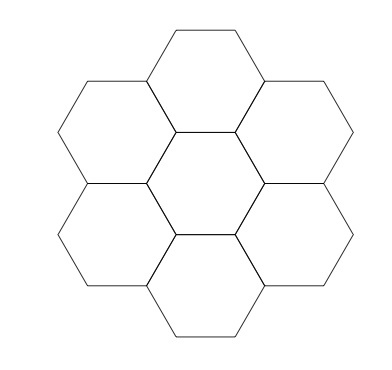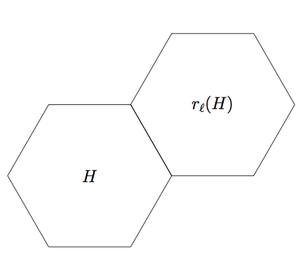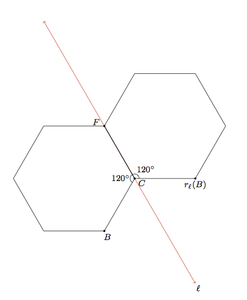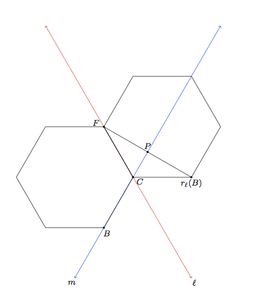# Building a tile pattern by reflecting hexagons

Alignments to Content Standards: G-CO.B.6

Below is a picture of a regular hexagon, which we denote by $H$, and two lines denoted $\ell$ and $m$, each containing one side of the hexagon:1. Draw $r_\ell(H)$, the reflection of the hexagon about $\ell$.
2. Draw $r_m(H)$, the reflection of the hexagon about line $m$, together with $H$ and $r_\ell(H)$.
3. Show that $H$ and its reflections about the six lines containing its sides make the following pattern:## IM Commentary

This task applies reflections to a regular hexagon to construct a pattern of six hexagons enclosing a seventh: the focus of the task is on using the properties of reflections to deduce this seven hexagon pattern. This pattern is also considered in 8.G Tile Patterns II: hexagons where the pattern is developed by studying angles. The teacher may wish to spend time having the students visualize the pattern of seven hexagons instead of providing the picture in part (c): this is particularly important if the students are not familiar with this pattern. Once the visualization has been accomplished, the focus of the task is on applying the definition of reflections to produce the pattern. In particular, in part (c) the central issue will be to show that $r_m(H)$ shares one side with $r_\ell(H)$ using the definition of reflections.

Reflections have a rather technical definition: if $\ell$ is a line and $P$ a point not on $\ell$ then the reflection of $P$ about $\ell$ is the point $r_\ell(P)$ so that $\ell$ is the perpendicular bisector of segment $\overline{Pr_\ell(P)}$. The instructor may wish to recall this definition and may wish to go over the argument from 8.G Tile Patterns II: hexagons to familiarize students with the seven hexagon configuration so they can focus on working with the definition of reflections.

The pattern of hexagons can be extended indefinitely in all directions: each outer hexagon in the seven hexagon configuration from part (c) can in turn be surrounded by six hexagons, using successive reflections. Repetition of this process generates the pattern seen here:

## Solution

1. The reflection of $H$ about $\ell$ is pictured below:The picture will be explained in detail in part (c).

2. The hexagon $H$ and its reflections about $\ell$ and $m$ are pictured here:This configuration will be carefully explained in part (c): the vital issue is to show that $r_\ell(H)$ and $r_m(H)$ share one side as pictured.

3. We begin by analyzing the reflection of $H$ about $\ell$ more closely. This is pictured below with some points labelled on $H$ and its reflection:The interior angle $BCF$ of the regular hexagon $H$ measures $120^\circ$. Since reflections preserve angle measures, angle $r_\ell(B)CF$ also measures $120^\circ$. This means that $\angle BCr_\ell(B)$ must also measure $120^\circ$ since it forms a full circle together with angles $BCF$ and $r_\ell(B)CF$. This will be important as we consider the reflection of $H$ over line $m$ joining vertices $B$ and $C$:Also pictured is the segment joining points $F$ and $r_\ell(B)$ and the point $P$ where $m$ meets this segment. We know that reflection about $m$ maps $B$ to $B$ and $C$ to $C$ because $B$ and $C$ both lie on $m$. We claim that reflection about $m$ maps $F$ to $r_\ell(B)$. For this it is sufficient to show that $m$ is the perpendicular bisector of segment $\overline{Fr_\ell(B)}$. We will show that this is true by showing that triangles $FPC$ and $r_\ell(B)PC$ are congruent: this will establish both that $\overline{FP}$ and $\overline{r_\ell(B)P}$ are congruent and that angles $FPC$ and $r_\ell(B)PC$ are right angles, making $m$ the perpendicular bisector of $\overline{Fr(B)}$ as desired.

Segment $\overline{FC}$ is congruent to segment $\overline{BC}$ since both are sides of a regular hexagon. Segment $\overline{r_\ell(B)C}$ is congruent to segment $\overline{BC}$ because reflections preserve distance. Therefore segment $\overline{FC}$ is congruent to segment $\overline{r_\ell(B)C}$. Segment $\overline{PC}$ is congruent to itself. We know that $m(\angle PCF) = 60$ because it is supplementary to the interior angle $BCF$ of a regular hexagon. We know $m(\angle FCr_\ell(B)) = 120$ because it is an interior angle of a regular hexagon. Therefore $$m(\angle PCr_\ell(B)) = m(\angle FCr_\ell(B)) - m(\angle(PCF)) = 120 - 60 = 60.$$ So angles and $FCP$ and $r_\ell(B)CP$ are congruent. By SAS, triangles $PCr_\ell(B)$ and $PCF$ are congruent.

We have now shown that reflection about $m$ maps $F$ to $r_\ell(B)$ and so maps $\overline{CF}$ to $\overline{Cr_\ell(B)}$ and so the picture for part (b) is correct. We may now continue this procedure, moving around sides of $H$ in a clockwise fashion, reflecting around the line containing each side. The order in which the reflected hexagons appear is shown in the picture below:At each step, the reflection of $H$ will share one side with the previously reflected hexagon: the explanation for part (b) above applies word for word in each case. When we come to the hexagon marked $6$, this reflected hexagon shares one side with the hexagon $5$ but it also shares a side with hexagon $1$. To see why, consider the following picture:If we apply the argument of part (b) to the reflections about $\ell^\prime$ and $m^\prime$ (in place of $\ell$ and $m$) this will show that the reflection of $H$ about $\ell^\prime$ (Hexagon 6) will share a side with the reflection of $H$ about $m^\prime$ (Hexagon 1). So the picture of a single regular hexagon surrounded by $6$ regular hexagons can be produced by reflecting $H$ about the $6$ lines containing its sides.